﻿ Design & Analysis of a Novel Rectangular Microstrip Patch Antenna with Improved Performance Using MATLAB for Pervasive Wireless Applications

### Design & Analysis of a Novel Rectangular Microstrip Patch Antenna with Improved Performance Usin...

Nishant Kumar, Ashutosh Kumar Pandey, Aditya Prakash, Shiv Mani Kumar, Rupali Bihari, Manas Ranjan JenaOPEN ACCESSPEER-REVIEWED

## Design & Analysis of a Novel Rectangular Microstrip Patch Antenna with Improved Performance Using MATLAB for Pervasive Wireless Applications

Nishant Kumar1, Ashutosh Kumar Pandey1, Aditya Prakash1, Shiv Mani Kumar1, Rupali Bihari1, Manas Ranjan Jena2,1B.Tech 4th Year, Dept. of ETC, SIET, Dhenkanal, Odisha, India

2Asst. Prof. Dept. of ETC, SIET, Dhenkanal, Odisha, India

### Abstract

In this paper, we have achieved an efficient rectangular patch antenna with optimized parameters by using MATLAB. The proposed antenna is a type of radio antenna with a low profile which is mounted on a flat surface. It consists of a flat rectangular sheet or patch of metal, mounted over a larger sheet of metal called a ground plane. A resonant frequency of 3 GHz is achieved with improved parameters like high return loss, Low VSWR, High directivity & gain, High efficiency. So the proposed antenna can be suitable for the radio astronomy & space research applications.

### At a glance: Figures

12
Prev Next

• Kumar, Nishant, et al. "Design & Analysis of a Novel Rectangular Microstrip Patch Antenna with Improved Performance Using MATLAB for Pervasive Wireless Applications." Wireless and Mobile Technologies 2.1 (2014): 7-11.
• Kumar, N. , Pandey, A. K. , Prakash, A. , Kumar, S. M. , Bihari, R. , & Jena, M. R. (2014). Design & Analysis of a Novel Rectangular Microstrip Patch Antenna with Improved Performance Using MATLAB for Pervasive Wireless Applications. Wireless and Mobile Technologies, 2(1), 7-11.
• Kumar, Nishant, Ashutosh Kumar Pandey, Aditya Prakash, Shiv Mani Kumar, Rupali Bihari, and Manas Ranjan Jena. "Design & Analysis of a Novel Rectangular Microstrip Patch Antenna with Improved Performance Using MATLAB for Pervasive Wireless Applications." Wireless and Mobile Technologies 2, no. 1 (2014): 7-11.

 Import into BibTeX Import into EndNote Import into RefMan Import into RefWorks

### 1. Introduction

Microstrip Patch antenna (MPA) is a new & exciting technology efficiently for application as conformal antennas on missiles & aircraft. MPA has found increasing use as it can be fabricated by lithographic circuits. The advantages of MPA at microwave frequencies is its compatibility with large scale printed circuit fabrication. MPA is finding increasing popularity owing to its advantages like less size, Less costly, Conformity to the supporting structure and ease of fabrication . To analyze its impendence and radiation properties many elaborate analytical techniques are proposed & used. Numerical methods are also developed & have received increasing attention in the recent years, these being primarily based on Sommerfeld-type integral equations. All these methods assume that the dielectric substrate & the supporting ground plane are infinite in extent . So the solution is valid for infinite geometries, or when the substrate and ground-plane dimensions are relatively large. The assumption does not introduce a severe difficulty in impendence calculations since microstrip geometries are inherently resonant structures and their impendence characteristic is primarily controlled by the printed elements .

When the microstrip geometry is non-circular, or even arbitrary in shape, one can use a surface-patch segmentation over the conducting and dielectric surfaces. The current and surface distributions can then be represented by appropriate basis functions over these patches to convert the integral equations to a matrix equation using a moment method. The solution of the resulting matrix equation give the surface distributions over the conducting and dielectric surfaces .

### 2. Motivation

Microstrip antennas received considerable attention starting in the 1970. The rectangular patch is by far the widely used configuration. It is very easy to analyze using both the transmission line and cavity models, which are most accurate for thin substrates .

A rectangular microstrip antenna can be represented as an array of two radiating narrow slots each of width W and height H, separated by a distance L. Basically the transmission line model represents the micristrip antenna by two slots, separated by a low-impedance Zc transmission line of length L .

Fringing Effect: The amount of fringing is a function of the dimension of patch and the height of the substrate. It is the ratio of the length of the patch L to the height h of the substrate(L/h) and the dielectric constant Er of the substrate.

For low frequency the effective dielectric constant is essentially constant. At intermediate frequencies its value begins to monotonically increase and eventually approach the values of the dielectric constant of the substrate . The initial values of the effective dielectric constant are given by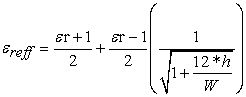The technique can be used effectively to match the patch antenna using a microstrip line feed whose characteristics impedance is given by-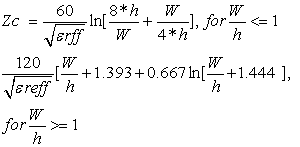Where,

W =Width of the microstrip line

εrff =Effective Dielectric Constant

h =Heigth of the microstrip line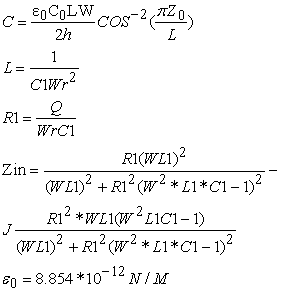Where,

C=Capacitance of patch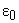=permitivity of vacuum

Q=Quality factor

L=Inductance of patch=Characteristic impedance of antenna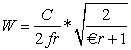### 3. Directivity

As for every other antenna, the directivity is one of the most important figure-of-merit whose definition is given by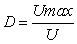.

The directivity of a single slot can be expressed asWhere, X =KW

### 4. Retur N Loss

Return loss is related to both standing wave ratio (SWR) and reflection coefficient (Γ). Increasing return loss corresponds to lower SWR. Return loss is a measure of how well devices or lines are matched. A match is good if the return loss is high. A high return loss is desirable and results in a lower insertion loss .

Return loss is used in modern practice in preference to SWR because it has better resolution for small values of reflected wave.### 5. VSWR

Voltage standing wave ration(VSWR) defined as the ration of the maximum to minimum voltage of the antenna. The reflection coefficient p is defined as a ration between incident wave amplitude Vi and reflected voltage wave amplitude Vd and by using the definition of a voltage coefficient at the input terminals of the antenna Г , as shown in belowWhere,

Zo =characteristics impedance of the antenna.

Zi = input impedance

If the equation is solved for the reflection coefficient, it is found that where the reflection coefficient p is the absolute value of magnitude of Г.consequently, VSWR=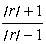The characteristics can be calculated as Zo =L = inductance of the antennaThen we can get the characteristics impedance is belowThe return loss S11 is related through the following equation,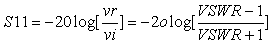Actual length of patch = Lff - 2∆L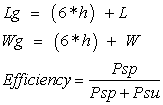Psp =The power radiated in space waves

Psu =The power radiated on surface waves

Psp+ Psu is then the total power delivered to the printed element.

5.1. Calculation of Efficiency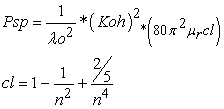Where,

C1 is constant that depends upon the substrate of material & n is the index refraction of the substrate.Putting C1 and after final calculation we get,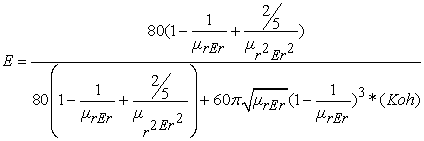5.2. Antenna Design Specification

The proposed antenna is designed by using following specifications. The dielectric constant(ε)=2.32, the substrate thickness (in mm) (h): 2.88, the frequency (GHz)(f): 2.4. The width of the patch is: 48.5094 mm, The length of the patch is: 39.5085 mm, The length of the ground plane is: 56.7885 mm, The width of the ground plane is: 65.7894 mm, The inset feed point is: 13.8467 mm, The conductance is: 0.0015208mho, The resonant input resistance is: 244.0171ohm, The input impedance is: 243.4299ohm, The output impedance is: 110.3245ohm.

The coaxial feed point x coordinate is: 8.9792 mm, The coaxial feed point y coordinate is: 24.2547 mm. The proposed antenna is designed by using MATLAB.

5.3. Simulation results & Result Analysis

Major simulated results are provided below & are summarized in the Table 1.

#### Table 1. various performance parameters of the proposed antennaDownload asPowerPoint Slide

Veiw figureView current table in a new window

### 6. Conclusion

The effect of curvature on the input impedance, return loss, voltage standing wave ratio of conformal microstrip antenna on cylindrical bodies for TM mode is studied in this paper. We have achieved A resonant frequency of 3 GHz with improved parameters like high return loss of -17.5908 dB, Low VSWR of 2.4186, High directivity of 7.1023 dBi & gain of 6.3342 dB, High efficiency of 83.7904%. So the proposed antenna can be suitable for the radio astronomy & space research applications.

### References

  J R James & P S Hall, “Handbook of Microstrip Antennas”, Peter Peregrinus Ltd, UK, 1989.In article  Balanis, Constantine, “Antenna theory-Analysis and Design”, John Wiley & Sons Ltd, Reprinted 2008.In article  I. J. Bahl and P. Bhartia, “Microstrip Antennas”, Artech House, Deldham, MA, 1980.In article  D.M. Pozar, “Microstrip Antennas,” Proc. IEEE, Vol. 80, No. 1, pp. 79-81, January 1992.In article CrossRef  C. A. Balanis, “Advanced Engineering Electromagnetics”, John Wiley & Sons, New York, 1989.In article  E.O. Hammerstad, “Equations for Microstrip Circuit Design,” Proc. Fifth European Microwave Conf., pp. 268-272, September 1975.In article  K. R. Karver and J. W. Mink. “Microstrip Antenna Technology,” IEEE Trans. Antennas Propagat., Vol. AP-29, No. 1. Pp. 2-24, January 1981.In article CrossRef  S L Mallikarjun, P M Hadalgi, “Single layer modified Rectangular Microstrip array Antenna for multiband & wideband applications”, Indian Journal of Radio & Space Physics, Vol 39, June 2010, PP 156-162.In article  Shiv Charan Puri & M.G.Tiary, “A Novel Quad Band Rectangular Microstrip Patch Antenna for Wireless Applications”, International Symposium on Devices MEMS, Intelligent Systems & Communication (ISDMISC) 2011.In article  Devdutt Sharma1 & Laxmi Shrivastava, “Design And Analysis of Co-Planar Wideband Microstrip Slot Antenna Using MATLAB”, IJETAE, Volume 3, Issue 6, June 2013.In article  Md. Maruf Ahamed, Kishore Bhowmik & Md. Shahidulla, “Rectangular Microstrip Patch Antenna at 2GHZ on Different Dielectric Constant for Pervasive Wireless Communication”, IJECE, Vol. 2, No. 3, June 2012, pp. 417-424.In article